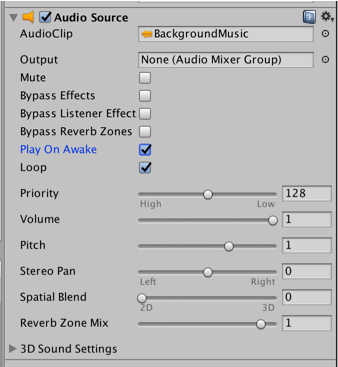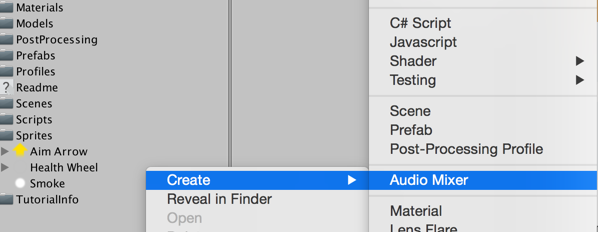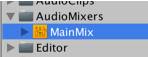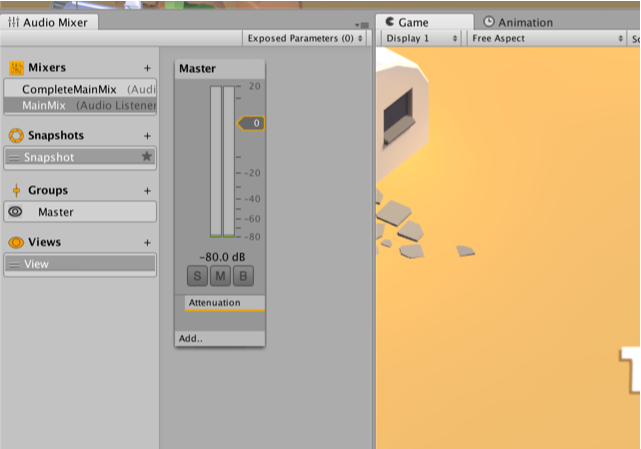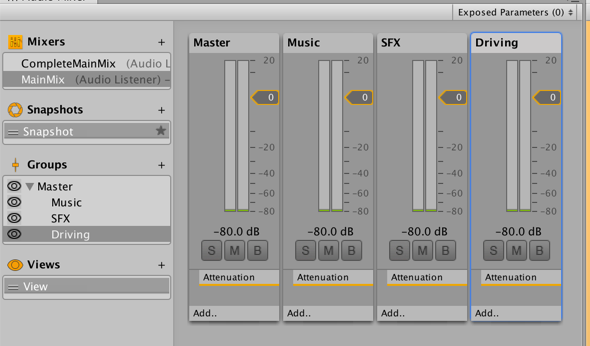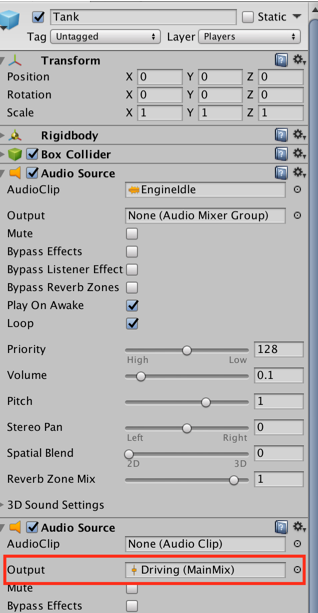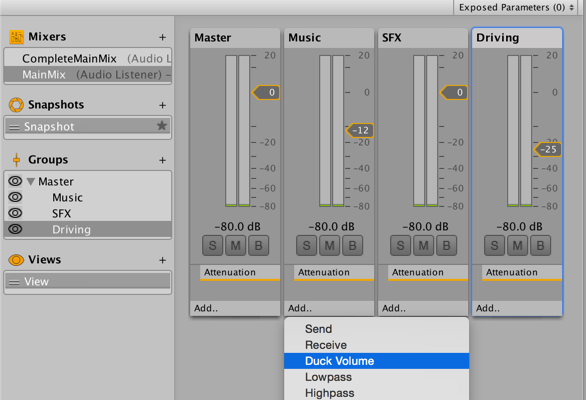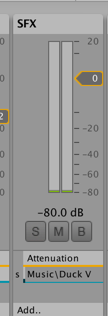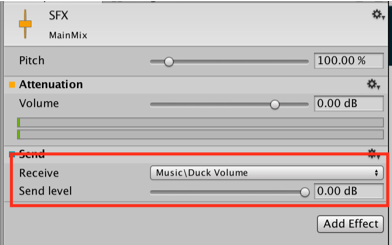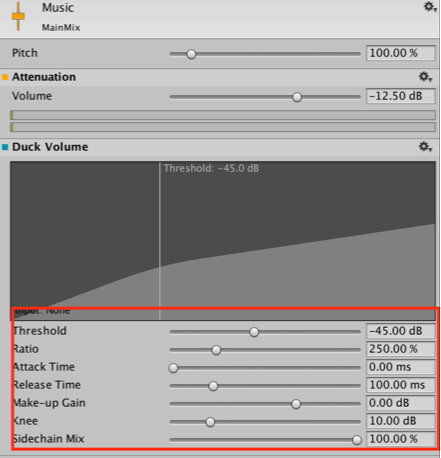# Scene Setup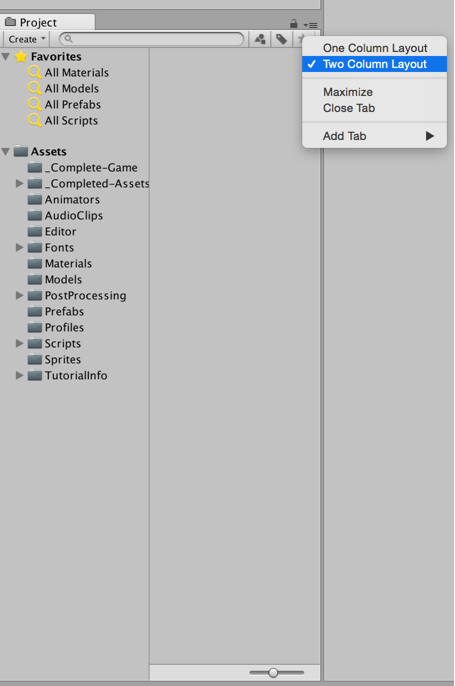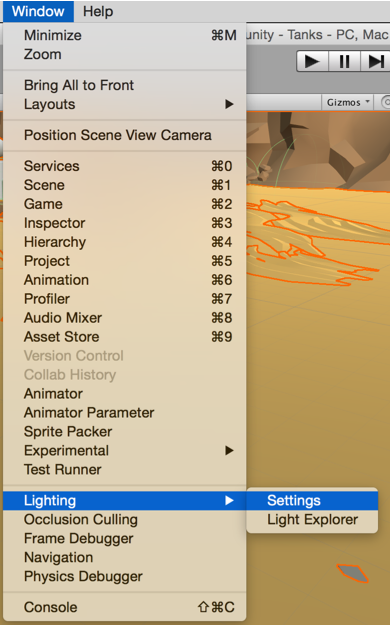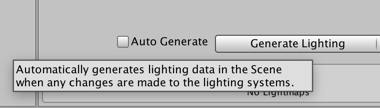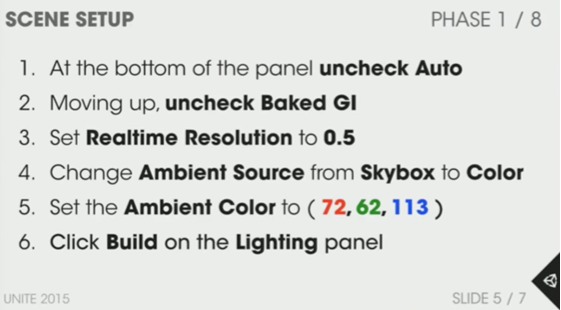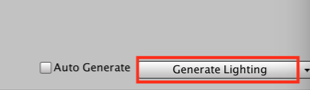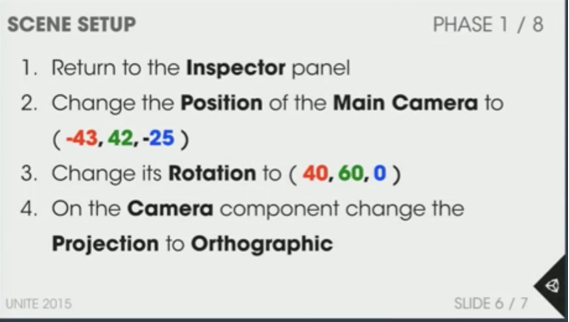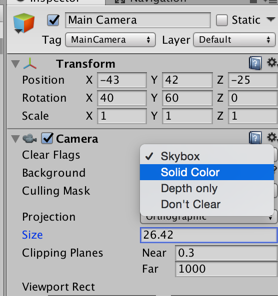# Tank Creation & Control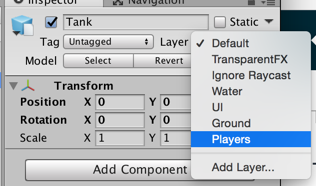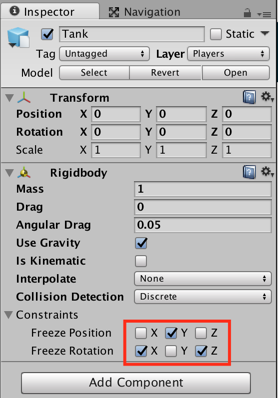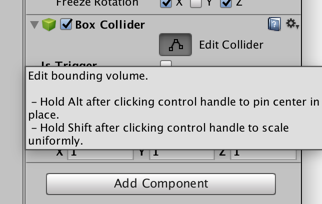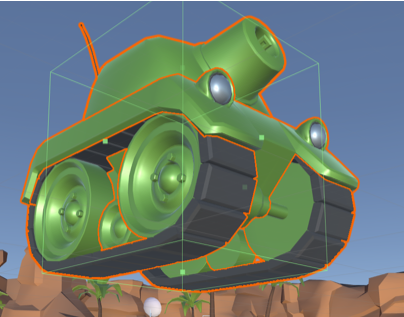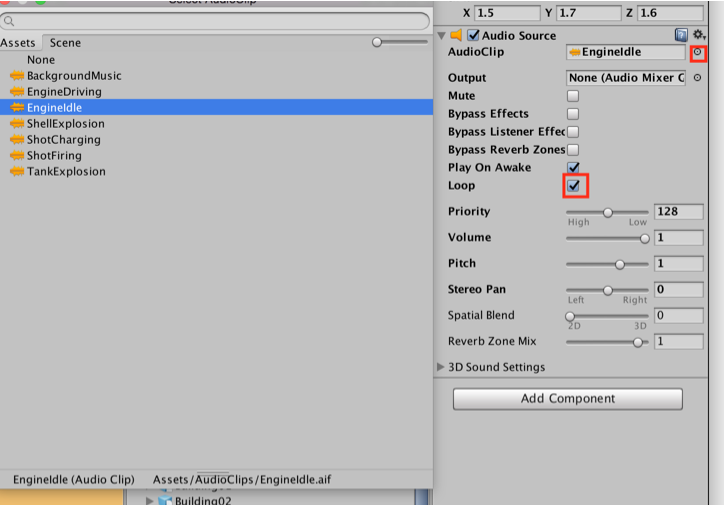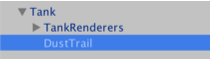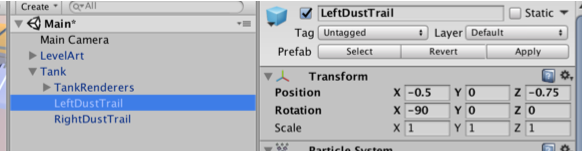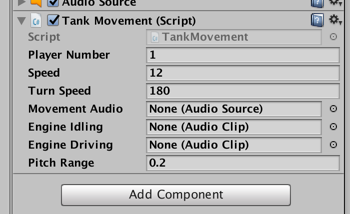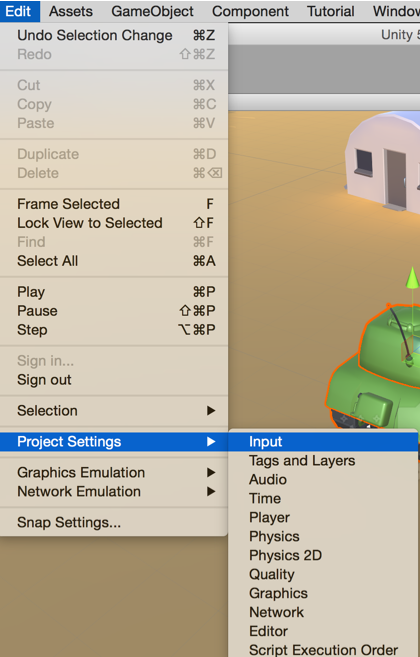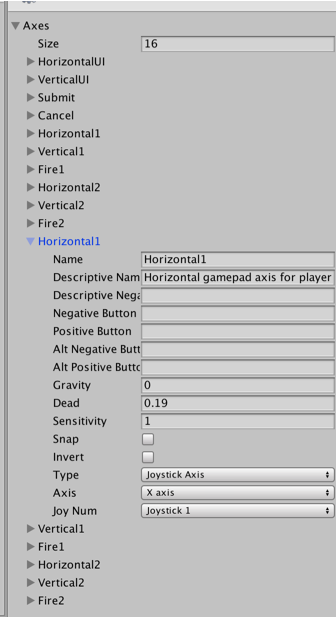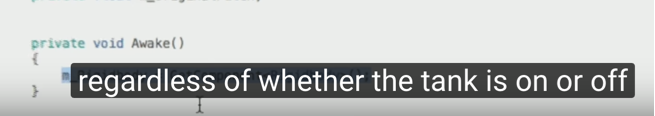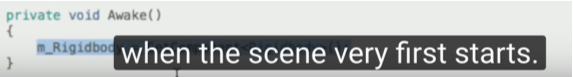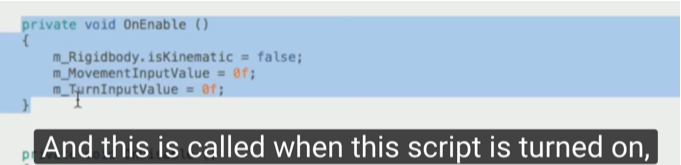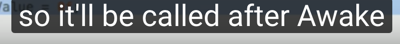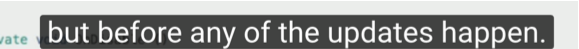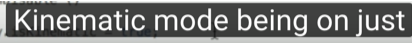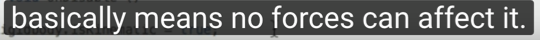``````private void EngineAudio()
{
// Play the correct audio clip based on whether or not the tank is moving and what audio is currently playing.
if (Mathf.Abs (m_MovementInputValue) < 0.1f && Mathf.Abs (m_TurnInputValue) < 0.1f) {
if (m_MovementAudio.clip == m_EngineDriving) {
m_MovementAudio.clip = m_EngineIdling;
m_MovementAudio.pitch = Random.Range (m_OriginalPitch - m_PitchRange, m_OriginalPitch + m_PitchRange);
m_MovementAudio.Play ();
}
} else {
if (m_MovementAudio.clip == m_EngineIdling)
{
m_MovementAudio.clip = m_EngineDriving;
m_MovementAudio.pitch = Random.Range (m_OriginalPitch - m_PitchRange, m_OriginalPitch + m_PitchRange);
m_MovementAudio.Play ();
}
}
}

private void FixedUpdate()
{
// Move and turn the tank.
Move();
Turn ();
}

private void Move()
{
Vector3 movement = transform.forward * m_MovementInputValue * m_Speed * Time.deltaTime;
m_Rigidbody.MovePosition(m_Rigidbody.position + movement);
}

private void Turn()
{
float turn = m_TurnInputValue * m_TurnSpeed * Time.deltaTime;
Quaternion turnRotation = Quaternion.Euler (0f, turn, 0f);
m_Rigidbody.MoveRotation (m_Rigidbody.rotation * turnRotation);
}``````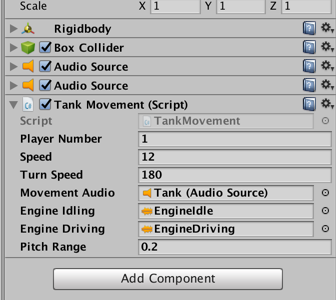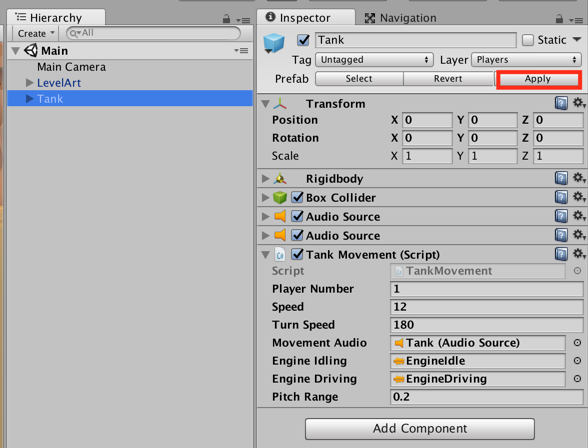# Camera Control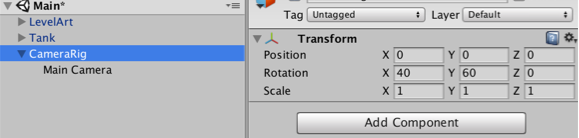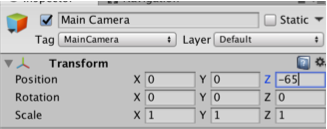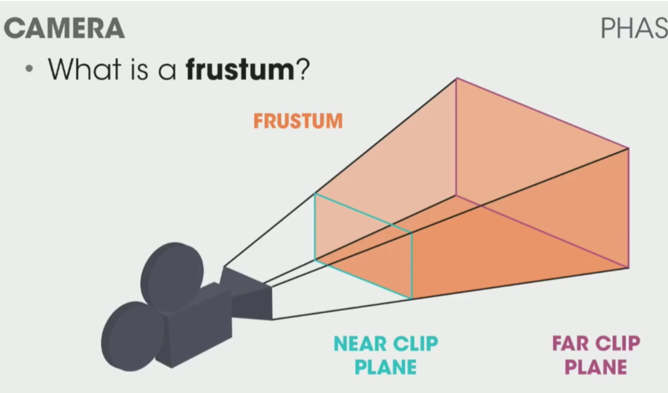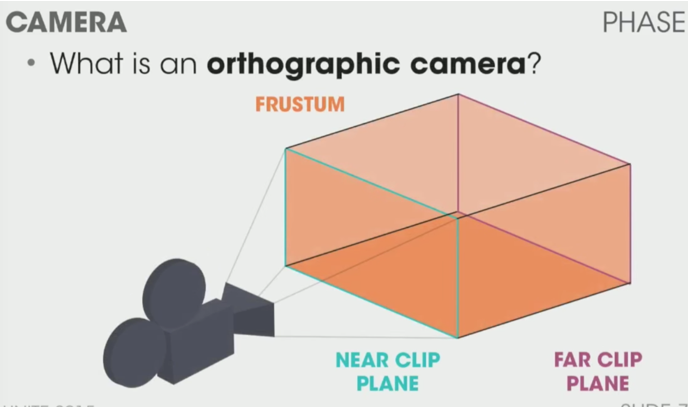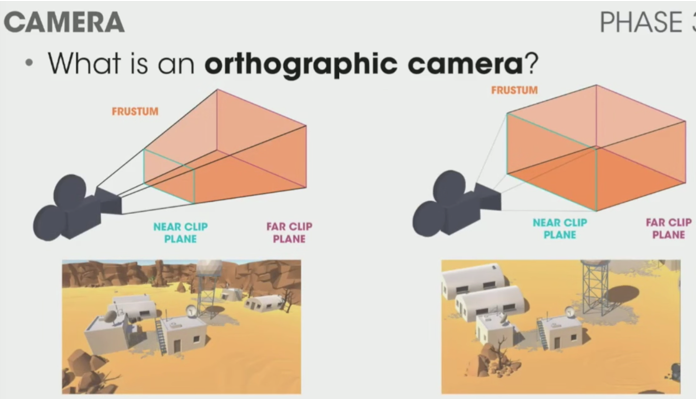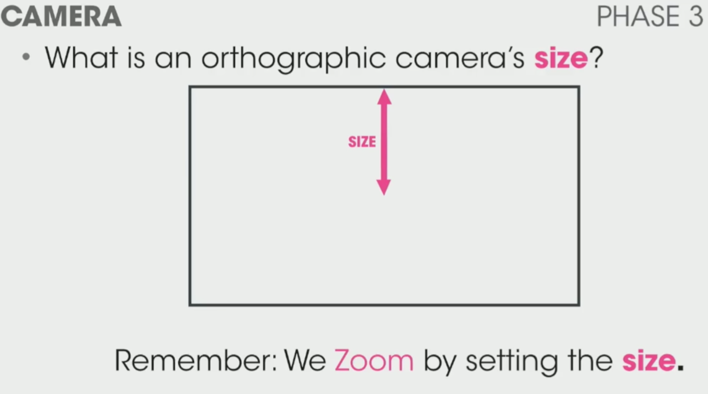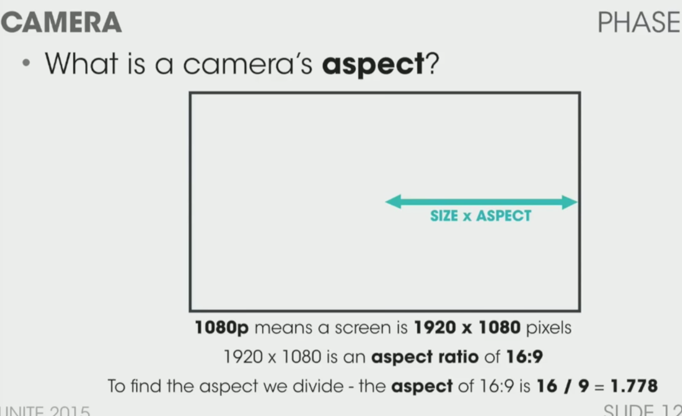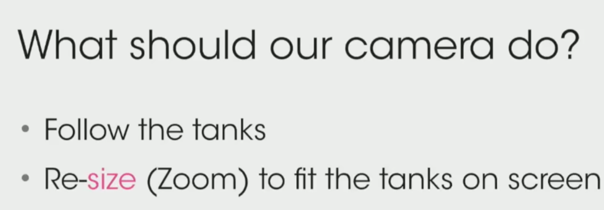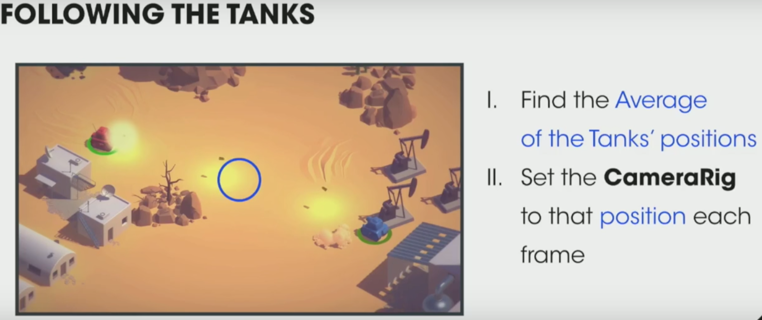``````[HideInInspector] public Transform[] m_Targets;

private void Move()
{
FindAveragePosition();
transform.position = Vector3.SmoothDamp(transform.position, m_DesiredPosition, ref m_MoveVelocity, m_DampTime);

}
private void FindAveragePosition()
{
Vector3 averagePos = new Vector3();
int numTargets = 0;

for (int i = 0; i < m_Targets.Length; i++)
{
if (!m_Targets[i].gameObject.activeSelf)
continue;
averagePos += m_Targets[i].position;
numTargets++;
}

if (numTargets > 0)
averagePos /= numTargets;
averagePos.y = transform.position.y;
m_DesiredPosition = averagePos;
}``````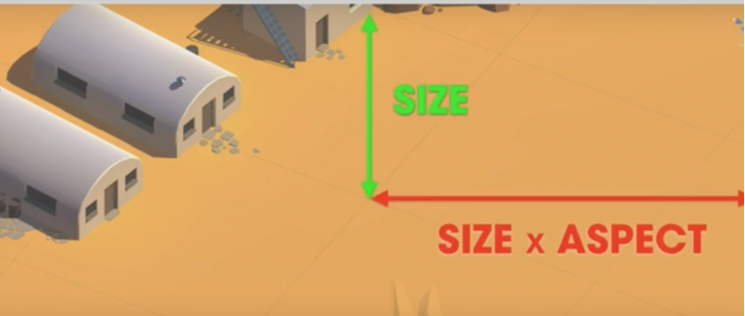``````private void Zoom()
{
float requiredSize = FindRequiredSize();
m_Camera.orthographicSize = Mathf.SmoothDamp(m_Camera.orthographicSize, requiredSize, ref m_ZoomSpeed, m_DampTime);
}

private float FindRequiredSize()
{
Vector3 desiredLocalPos = transform.InverseTransformPoint(m_DesiredPosition);
float size = 0f;
for (int i = 0; i < m_Targets.Length; i++)
{
if (!m_Targets[i].gameObject.activeSelf)
continue;

Vector3 targetLocalPos = transform.InverseTransformPoint(m_Targets[i].position);
Vector3 desiredPosToTarget = targetLocalPos - desiredLocalPos;
size = Mathf.Max (size, Mathf.Abs (desiredPosToTarget.y));
size = Mathf.Max (size, Mathf.Abs (desiredPosToTarget.x) / m_Camera.aspect);
}
size += m_ScreenEdgeBuffer;
size = Mathf.Max(size, m_MinSize);
return size;
}
``````
• `InverseTransformPoint` : Transforms position from world space to local space.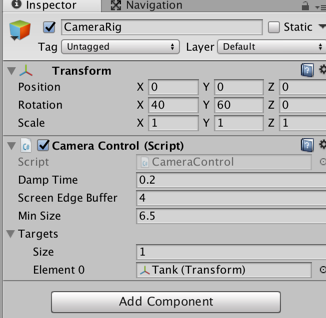# Tank Health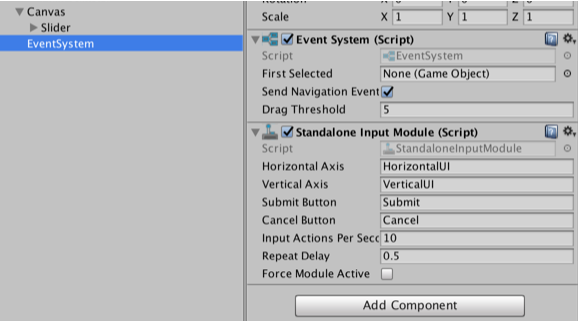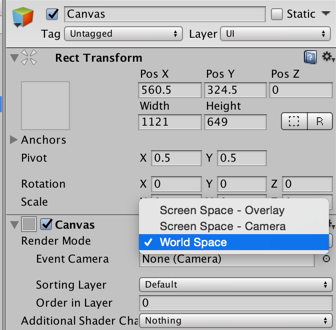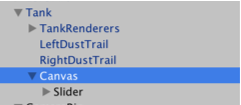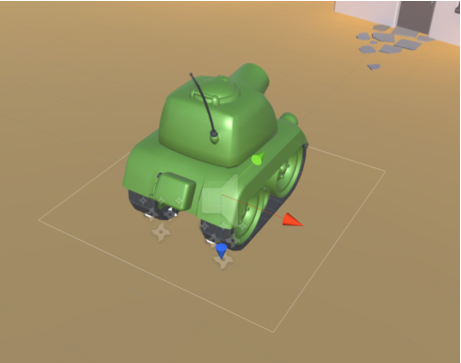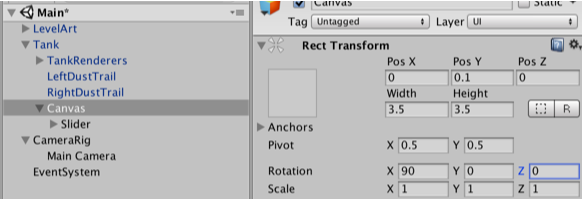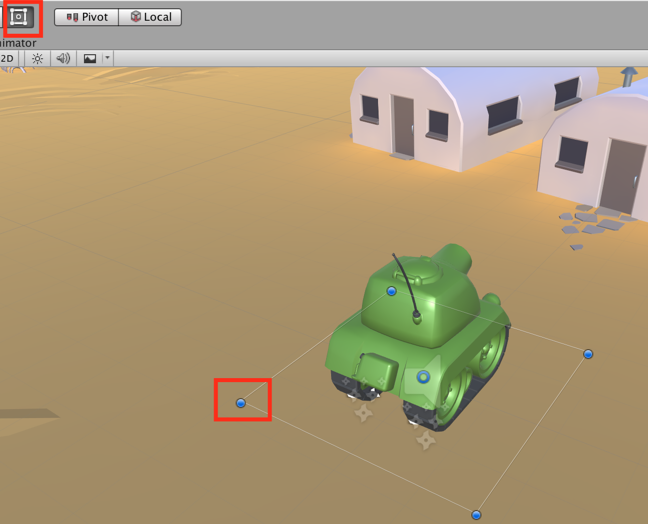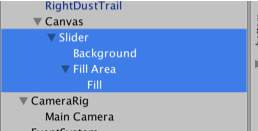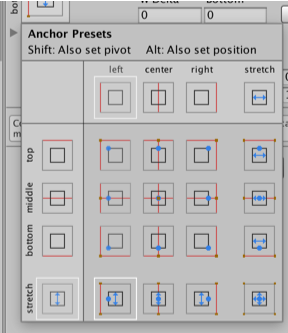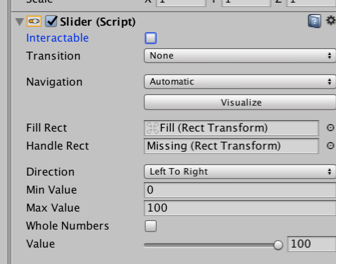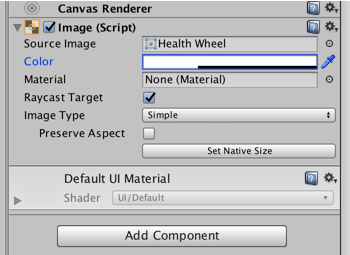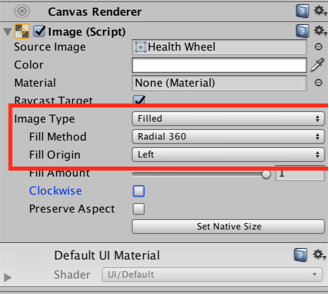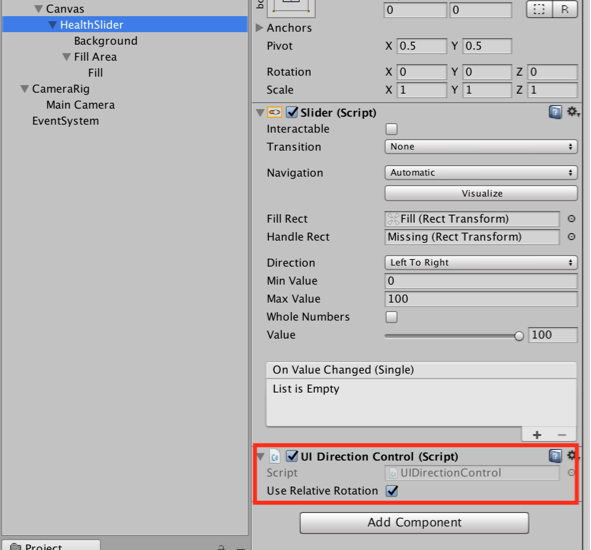``````using UnityEngine;

public class UIDirectionControl : MonoBehaviour
{
public bool m_UseRelativeRotation = true;

private Quaternion m_RelativeRotation;

private void Start()
{
m_RelativeRotation = transform.parent.localRotation;
}

private void Update()
{
if (m_UseRelativeRotation)
transform.rotation = m_RelativeRotation;
}
}``````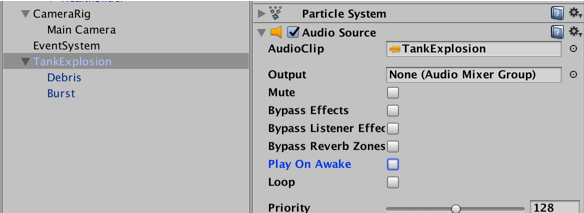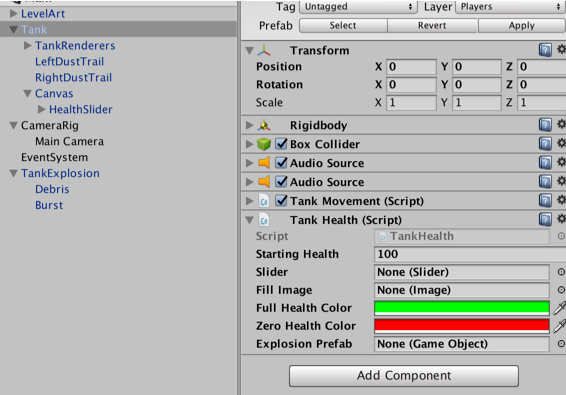``````using UnityEngine;
using UnityEngine.UI;

public class TankHealth : MonoBehaviour
{
public float m_StartingHealth = 100f;
public Slider m_Slider;
public Image m_FillImage;
public Color m_FullHealthColor = Color.green;
public Color m_ZeroHealthColor = Color.red;
public GameObject m_ExplosionPrefab;

private AudioSource m_ExplosionAudio;
private ParticleSystem m_ExplosionParticles;
private float m_CurrentHealth;
private bool m_Dead;

private void Awake()
{
m_ExplosionParticles = Instantiate(m_ExplosionPrefab).GetComponent<ParticleSystem>();
m_ExplosionAudio = m_ExplosionParticles.GetComponent<AudioSource>();

m_ExplosionParticles.gameObject.SetActive(false);
}

private void OnEnable()
{
m_CurrentHealth = m_StartingHealth;
m_Dead = false;

SetHealthUI();
}

public void TakeDamage(float amount)
{
m_CurrentHealth -= amount;

SetHealthUI ();

if (m_CurrentHealth <= 0f && !m_Dead) {
OnDeath ();
}
}

private void SetHealthUI()
{
m_Slider.value = m_CurrentHealth;
m_FillImage.color = Color.Lerp (m_ZeroHealthColor, m_FullHealthColor, m_CurrentHealth / m_StartingHealth);
}

private void OnDeath()
{
m_Dead = true;
m_ExplosionParticles.transform.position = transform.position;
m_ExplosionParticles.gameObject.SetActive (true);
m_ExplosionParticles.Play ();
m_ExplosionAudio.Play ();
gameObject.SetActive (false);
}
}``````

# Shell Creation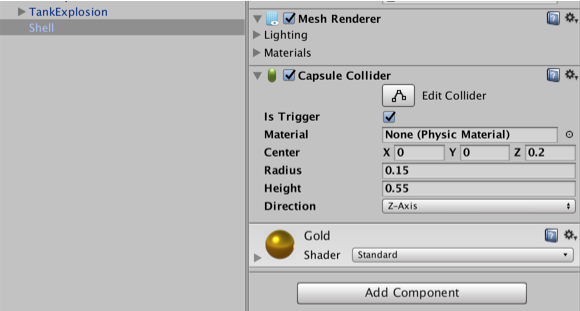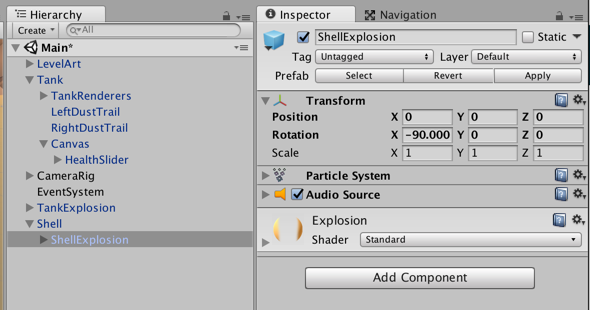``````using UnityEngine;

public class ShellExplosion : MonoBehaviour
{
public LayerMask m_TankMask;
public ParticleSystem m_ExplosionParticles;
public AudioSource m_ExplosionAudio;
public float m_MaxDamage = 100f;
public float m_ExplosionForce = 1000f;
public float m_MaxLifeTime = 2f;
public float m_ExplosionRadius = 5f;

private void Start()
{
Destroy(gameObject, m_MaxLifeTime);
}

private void OnTriggerEnter(Collider other)
{
Collider[] colliders = Physics.OverlapSphere (transform.position, m_ExplosionRadius, m_TankMask);

for (int i = 0; i < colliders.Length; i++)
{
Rigidbody targetRigidbody = colliders[i].GetComponent<Rigidbody> ();
if (!targetRigidbody)
continue;

targetRigidbody.AddExplosionForce (m_ExplosionForce, transform.position, m_ExplosionRadius);
TankHealth targetHealth = targetRigidbody.GetComponent<TankHealth> ();

if (!targetHealth)
continue;

float damage = CalculateDamage (targetRigidbody.position);
targetHealth.TakeDamage (damage);
}

// Unparent because the shell is gonna be destoryed
m_ExplosionParticles.transform.parent = null;
m_ExplosionParticles.Play();
m_ExplosionAudio.Play();

// Once the particles have finished, destroy the gameobject they are on.
Destroy (m_ExplosionParticles.gameObject, m_ExplosionParticles.duration);
// Destroy Shell
Destroy (gameObject);
}

private float CalculateDamage(Vector3 targetPosition)
{
Vector3 explosionToTarget = targetPosition - transform.position;
float explosionDistance = explosionToTarget.magnitude;
float relativeDistance = (m_ExplosionRadius - explosionDistance) / m_ExplosionRadius;
float damage = relativeDistance * m_MaxDamage;

damage = Mathf.Max (0f, damage);
return damage;
}
}``````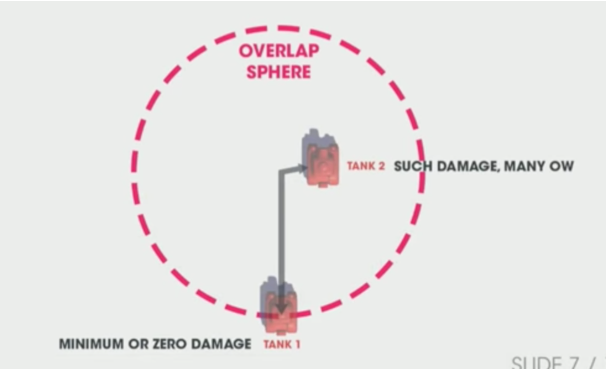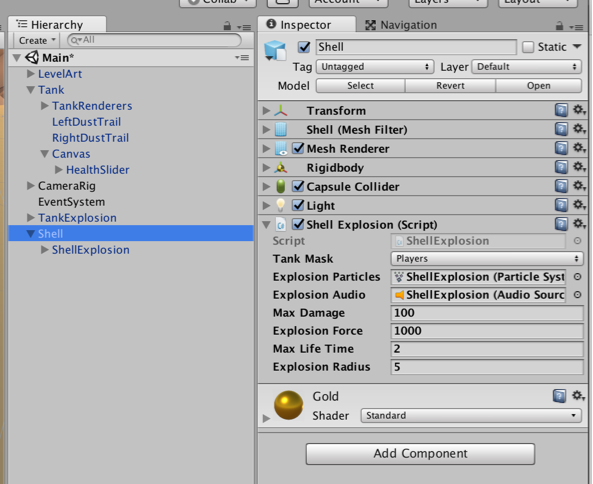# Firing Shells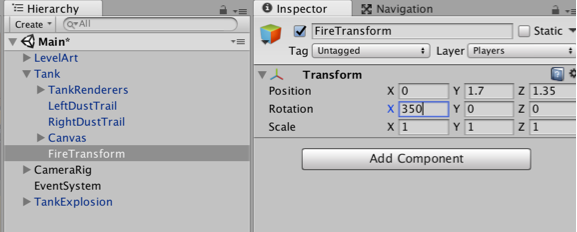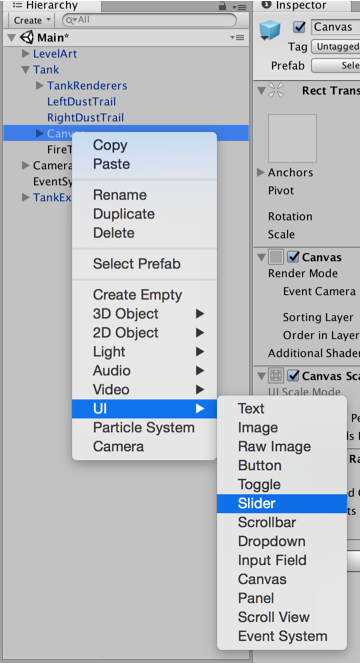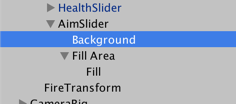• Click with `Alt` to fully open the hierarchy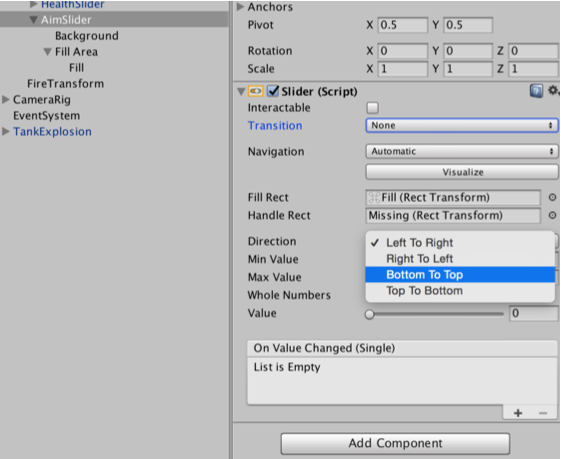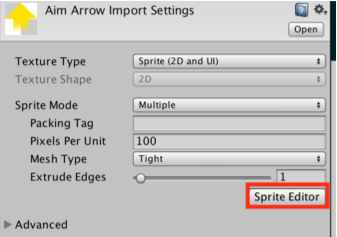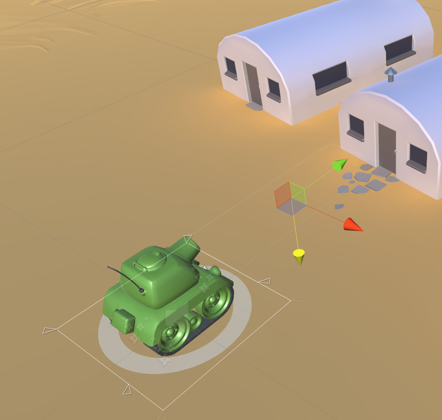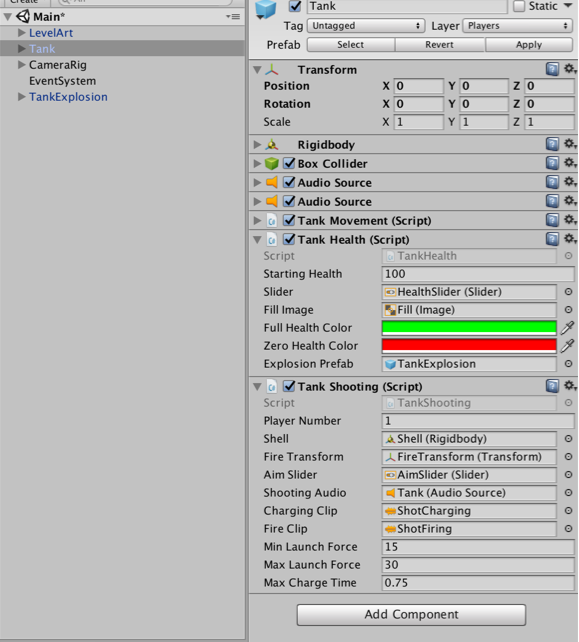# Game Managers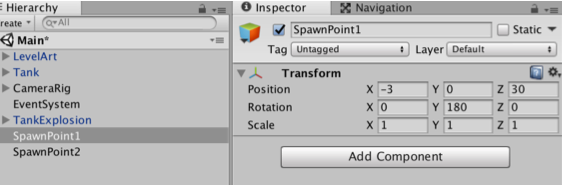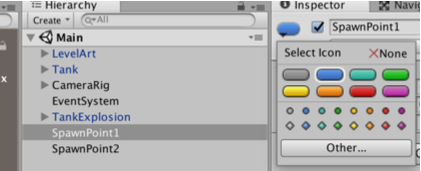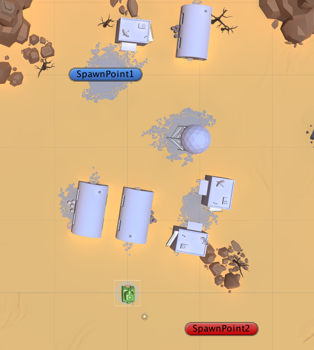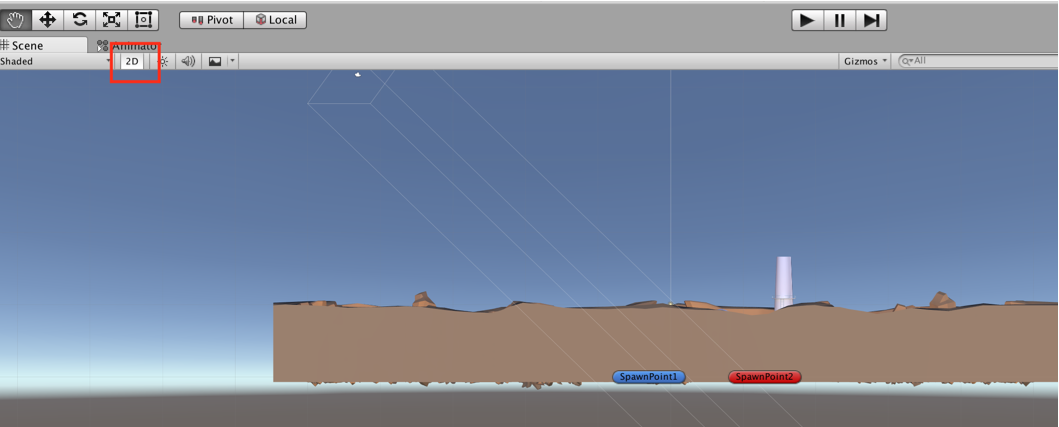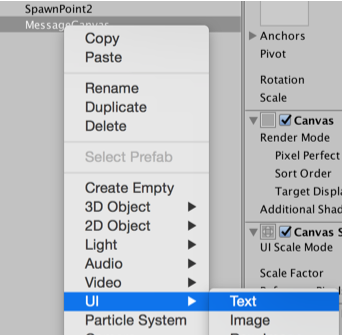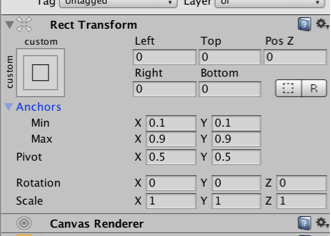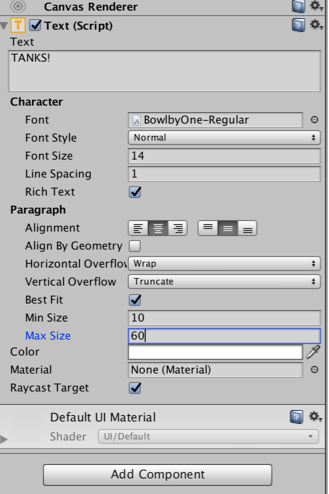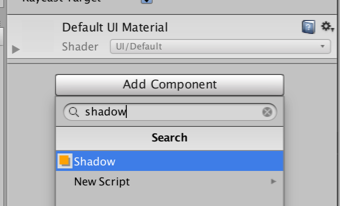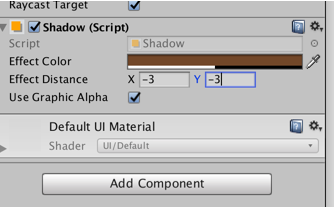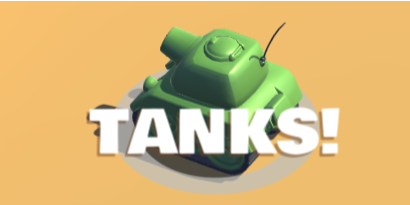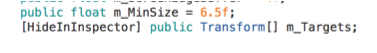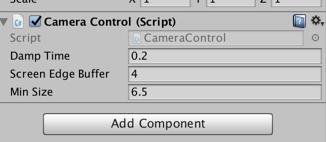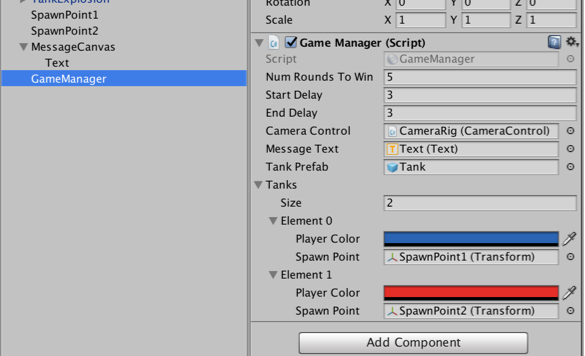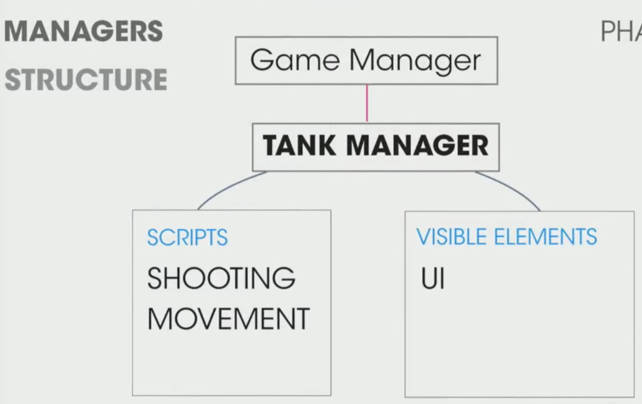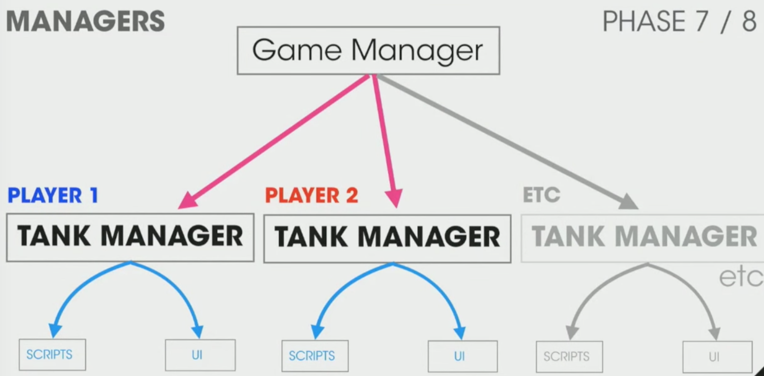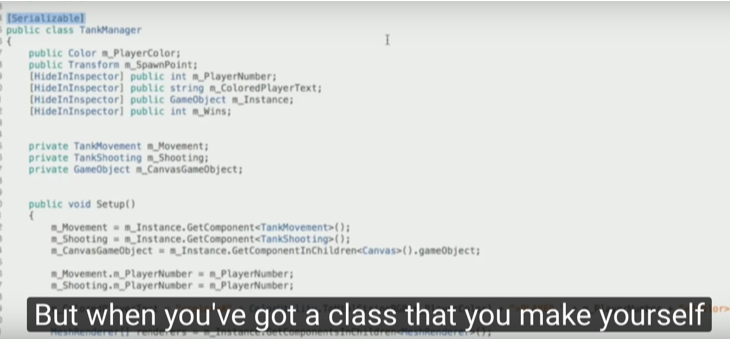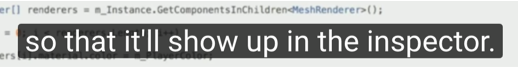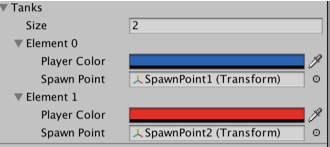TankManager:

``````public void Setup()
{
m_Movement = m_Instance.GetComponent<TankMovement>();
m_Shooting = m_Instance.GetComponent<TankShooting>();
m_CanvasGameObject = m_Instance.GetComponentInChildren<Canvas>().gameObject;

m_Movement.m_PlayerNumber = m_PlayerNumber;
m_Shooting.m_PlayerNumber = m_PlayerNumber;

m_ColoredPlayerText = "<color=#" + ColorUtility.ToHtmlStringRGB(m_PlayerColor) + ">PLAYER " + m_PlayerNumber + "</color>";

MeshRenderer[] renderers = m_Instance.GetComponentsInChildren<MeshRenderer>();

for (int i = 0; i < renderers.Length; i++)
{
renderers[i].material.color = m_PlayerColor;
}
}``````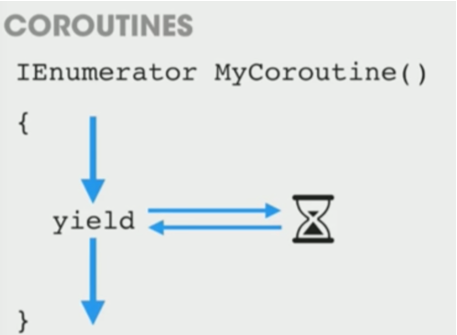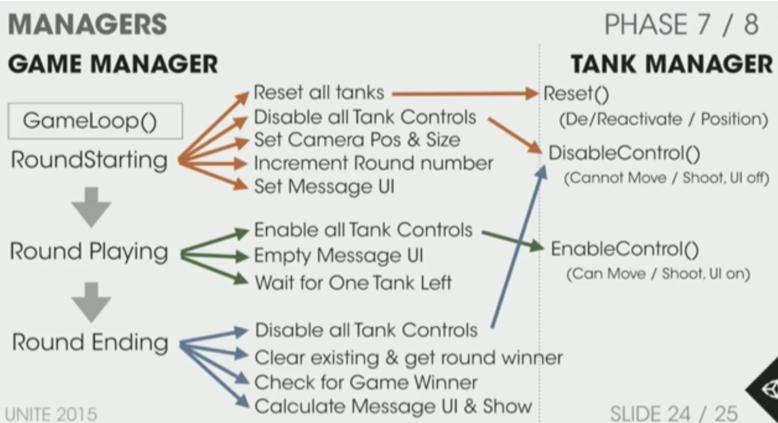GameManager:

``````private void Start()
{
m_StartWait = new WaitForSeconds(m_StartDelay);
m_EndWait = new WaitForSeconds(m_EndDelay);

SpawnAllTanks();
SetCameraTargets();

StartCoroutine(GameLoop());
}

private IEnumerator GameLoop()
{
yield return StartCoroutine(RoundStarting());
yield return StartCoroutine(RoundPlaying());
yield return StartCoroutine(RoundEnding());

if (m_GameWinner != null)
{
Application.LoadLevel (Application.loadedLevel);
}
else
{
StartCoroutine(GameLoop());
}
}

private IEnumerator RoundStarting()
{
ResetAllTanks ();
DisableTankControl ();
m_CameraControl.SetStartPositionAndSize ();

m_RoundNumber += 1;
m_MessageText.text = "ROUND " + m_RoundNumber;

yield return m_StartWait;
}

private IEnumerator RoundPlaying()
{
EnableTankControl ();
m_MessageText.text = string.Empty;

while (!OneTankLeft ()) {
yield return null;
}
}

private IEnumerator RoundEnding()
{
DisableTankControl ();
m_RoundWinner = null;
m_RoundWinner = GetRoundWinner ();
if (m_RoundWinner != null)
m_RoundWinner.m_Wins++;

m_GameWinner = GetGameWinner ();

string message = EndMessage ();
m_MessageText.text = message;

yield return m_EndWait;
}``````

# Audio Mixing